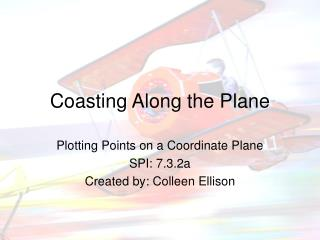DownloadDownload PresentationCoasting Along the Plane

# Coasting Along the Plane

Télécharger la présentation## Coasting Along the Plane

- - - - - - - - - - - - - - - - - - - - - - - - - - - E N D - - - - - - - - - - - - - - - - - - - - - - - - - - -
##### Presentation Transcript

1. Coasting Along the Plane Plotting Points on a Coordinate Plane SPI: 7.3.2a Created by: Colleen Ellison

2. Definition of Coordinate Plane • Coordinate plane is a plane formed by the intersection of a horizontal number line with a vertical number line. They intersect at their zero points. This point of intersection is called the origin  and written as (0, 0). • On a coordinate plane, the horizontal number line is called the x-axis and the vertical number line is called the y-axis.

3. WHAT! • Coordinate Plane- these are big words that simply mean something that can be located anywhere in time and space. • We use coordinate planes to create video games, design computer graphic programs, and to plot our place in the air or on the ocean • When plotting ordered pairs, the first number in parentheses is the number on the x-axis and the second number is on the y-axis. For example, the ordered pair (2,5); 2 is located on the x-axis and 5 is located on the y-axis.

4. It’s Really Very Easy! • Solved Example on Coordinate PlaneName the point having the coordinates (- 2.5, 2.5) on the coordinate plane. Choices: B E C G

5. On Your Own • Plot the following coordinates: • (2, -4) • (-3, 7) • (9, 0) • (0, -12)

6. Related Terms for Coordinate Plane • x-axis • y-axis • Plane • Ordered Pair • Coordinates • Origin • Intersection • Number Line

7. Resources: • The following websites have many fun and exciting activities for the teacher and tutorials for the student. http://themathworksheetsite.com www.dillustrated.com www.mathplayground.com www.mathtrain.com